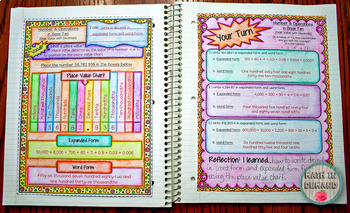### 5th Grade Math Interactive Notebook with Guided Notes and Examples

This listing is for my 5th grade math interactive notebook. The notebook has the same template as my other popular notebooks. There are 2 pages for each concept. The first page introduces the concept and provides notes. The second page is "Your Turn" where students will perform practice problems, and demonstrate their newly acquired knowledge.The notebook includes 5 units:

❶ Unit 1 – Operations & Algebraic Thinking

❷ Unit 2 – Number & Operations in Base Ten

➌ Unit 3 – Number & Operations–Fractions

❹ Unit 4 – Measurement & Data

❺ Unit 5 – GeometryThe breakdown of each unit is given below.

Unit 1 – Operations & Algebraic Thinking

★ Evaluating Expressions (Containing Parentheses, Brackets, or Braces)

★ Writing Expressions Containing Numerals and Symbols

★ Translating Expressions Containing Parentheses

★ Graphing Patterns (Graphs of Rules)

★ Writing Expressions from a Table of Values (Determining Relationship between Input and Output)

Unit 2 – Number & Operations in Base Ten

★ Digit Value with Decimals

★ Comparing Digit Place Values

★ Multiplying and Dividing in Base Ten

★ Word Problems in Base Ten

★ Multiples of 10, 100, and 1000

★ Read, write, and compare decimals to thousandths

★ Expanded Notation to Numeric Form

★ Numeric Form to Expanded Notation

★ Rounding Decimals

★ Multiplying Multi-Digit Whole Numbers

★ Whole Number Quotients

★ Dividing Decimals

★ Multiplying Decimals

Unit 3 – Number & Operations - Fractions

★ Adding & Subtracting Fractions with Mixed Numbers

★ Adding & Subtracting Fractions Word Problems

★ Multiplying Fractions

★ Multiplying Fractions with a Whole Number

★ Multiplying Fractions Word Problems

★ Area of a Rectangle with Fractional Lengths

★ Size Comparison of Fractions

★ Dividing Fractions by a Whole Number & Vice Versa

★ Dividing Fractions Word Problems

Unit 4 – Measurement & Data

★ Metric Units

★ Customary Units

★ Creating Line Plots

★ Intro to Volume

★ Intro to Unit Cube (Measuring Volume using Unit Cubes)

★ Calculating Volume of Rectangular Prisms

Unit 5 – Geometry

★ Intro to Coordinate System

★ Graphing Points from a Table

★ Classifying Shapes (Given Pictures)Connect with Me!

This resource includes a limited use license from Math in Demand. You may only use the resource for personal classroom use. This purchase does not allow you to transfer my resource to another teacher, school, or etc. You must purchase an additional license at a discounted cost.

Questions? Please email me at mathindemand@hotmail.com or click the Q&A tab.